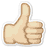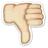Meaning Median age
What does Median age mean? Here you find 9 meanings of the word Median age. You can also add a definition of Median age yourself

# 1

10# Median age

The median age of a population is the age that divides the population into two numerically equal groups.
 Source: population-europe.eu

# 2

00# Median age

The age that divides a population into two numerically equal groups; that is, half the people are younger than this age and half are older.
 Source: prb.org

# 3

11# Median age

The median divides the age distribution into two equal parts: one-half of the cases falling below the median age and one-half above the median.
 Source: toxmap.nlm.nih.gov

# 4

11# Median age

Age that divides the population in two parts of equal size, that is, there are as many persons with ages above the median as there are with ages below the median.
 Source: esa.un.org

# 5

00# Median age

The median age of all people in a given geographic area.
 Source: carto.com

# 6

00# Median age

Age “x”, such that exactly one half of the population is older than “x” and the other half is younger than “x”.
 Source: finance.alberta.ca

# 7

00# Median age

The age at which 50% of the population is younger and 50% is older. Source: US Census Bureau.
 Source: oregonexplorer.info

# 8

11# Median age

Median age at diagnosis is the middle value, i.e. 50% of cancer cases are diagnosed at an older age and 50% at a younger age compared to the median age.
 Source: stats.health.act.gov.au

# 9

01# Median age

The median age is the age that divides the distribution of the population into two, such that half the population is below this age and half is above it.
 Source: statistics.gov.my

 Add meaning of Median age Word count:
 Name: E-mail: (* optional)

 << ICE DAM Boiling Temperature >>

Dictionary.university is a dictionary written by people like you and me.